# Selina Solutions Concise Maths Class 10 Chapter 14 Equation of a Line Exercise 14(E)

The last exercise has problems covering all the concepts discussed in this chapter. In order to get a strong overall understanding of this chapter, Selina Solutions for Class 10 Maths is the right tool. Students can use this for any quick references or doubt clearance, as per their convenience. The answers of this exercise can be accessed in the Selina Solutions Concise Maths Class 10 Chapter 14 Equation of a Line Exercise 14(E) PDF, in the link given below.

## Selina Solutions Concise Maths Class 10 Chapter 14 Equation of a Line Exercise 14(E) Download PDF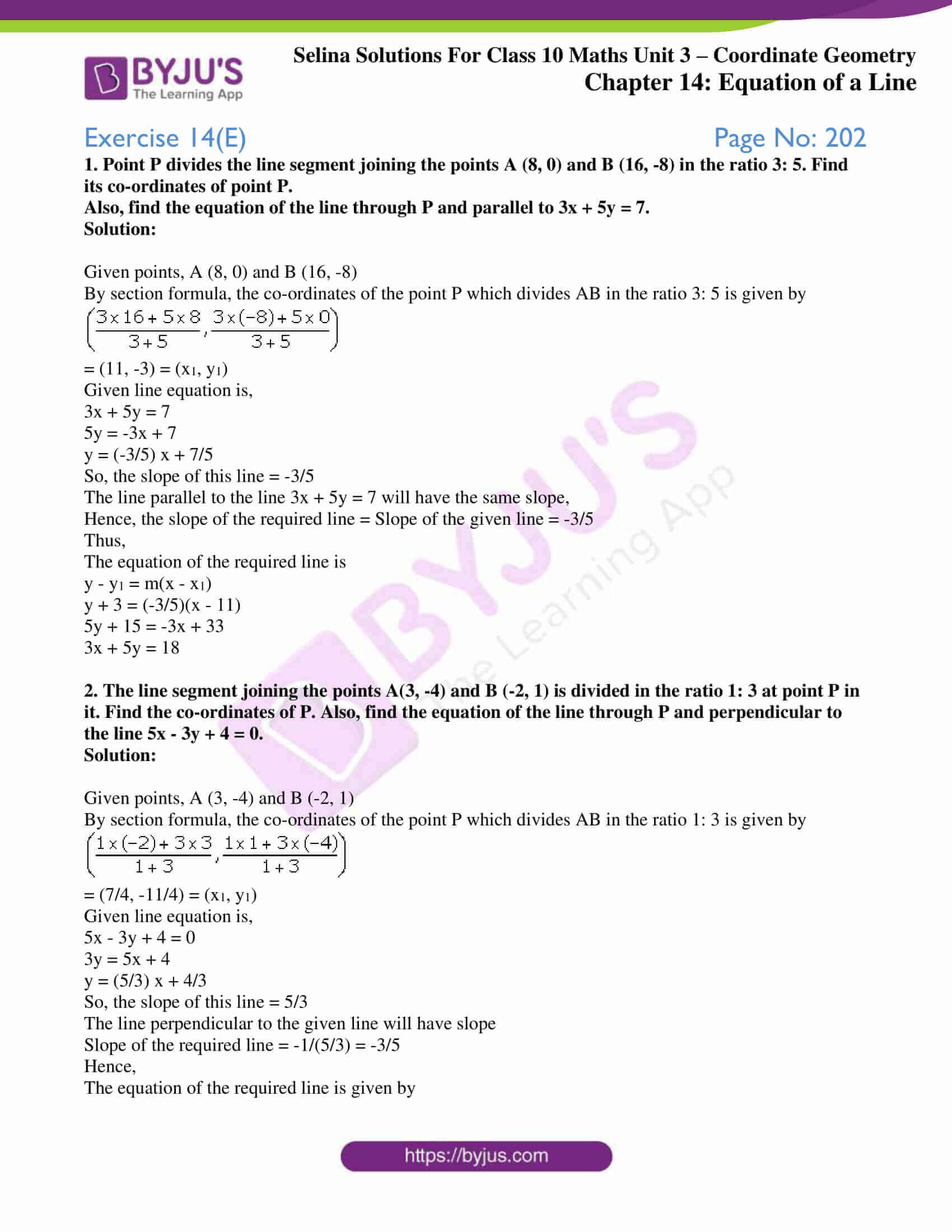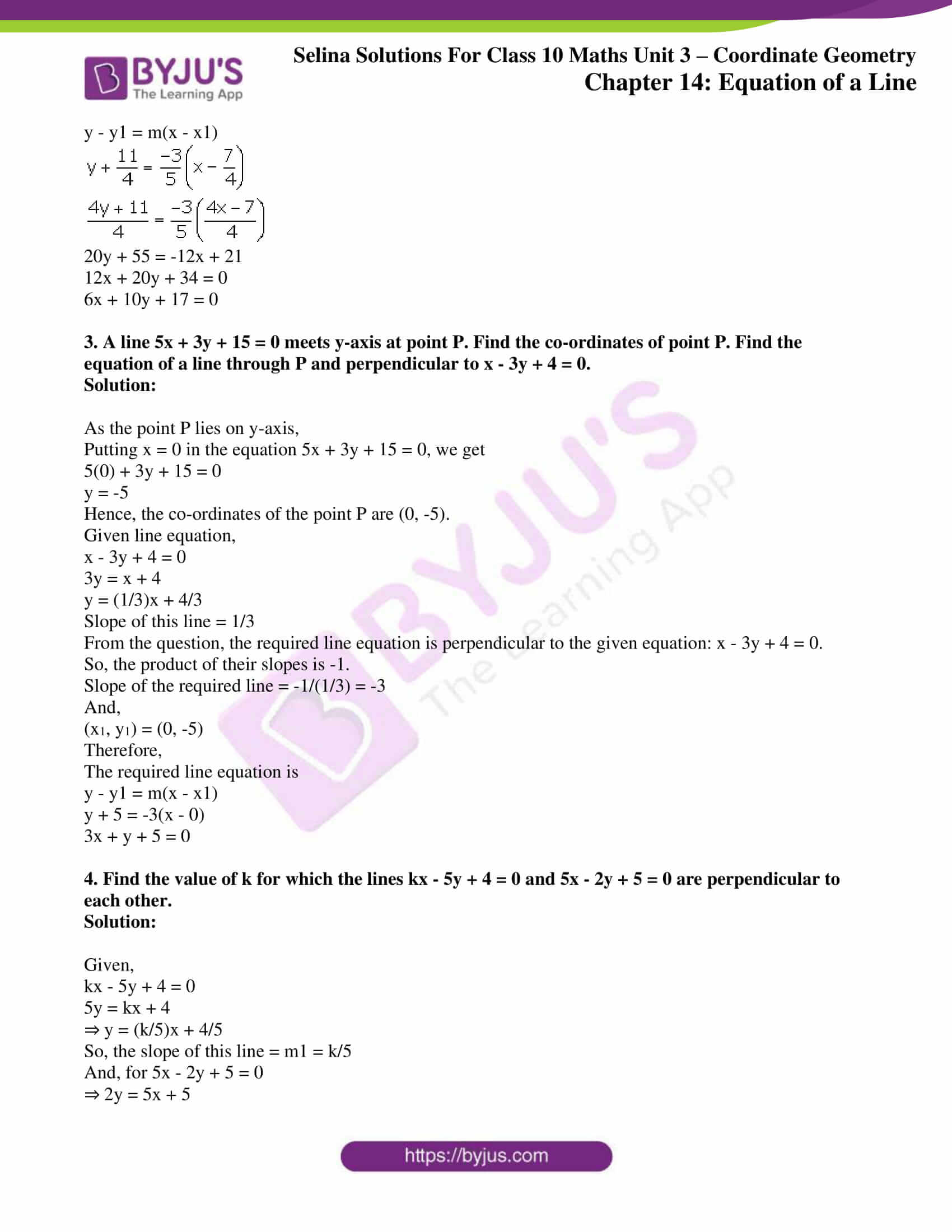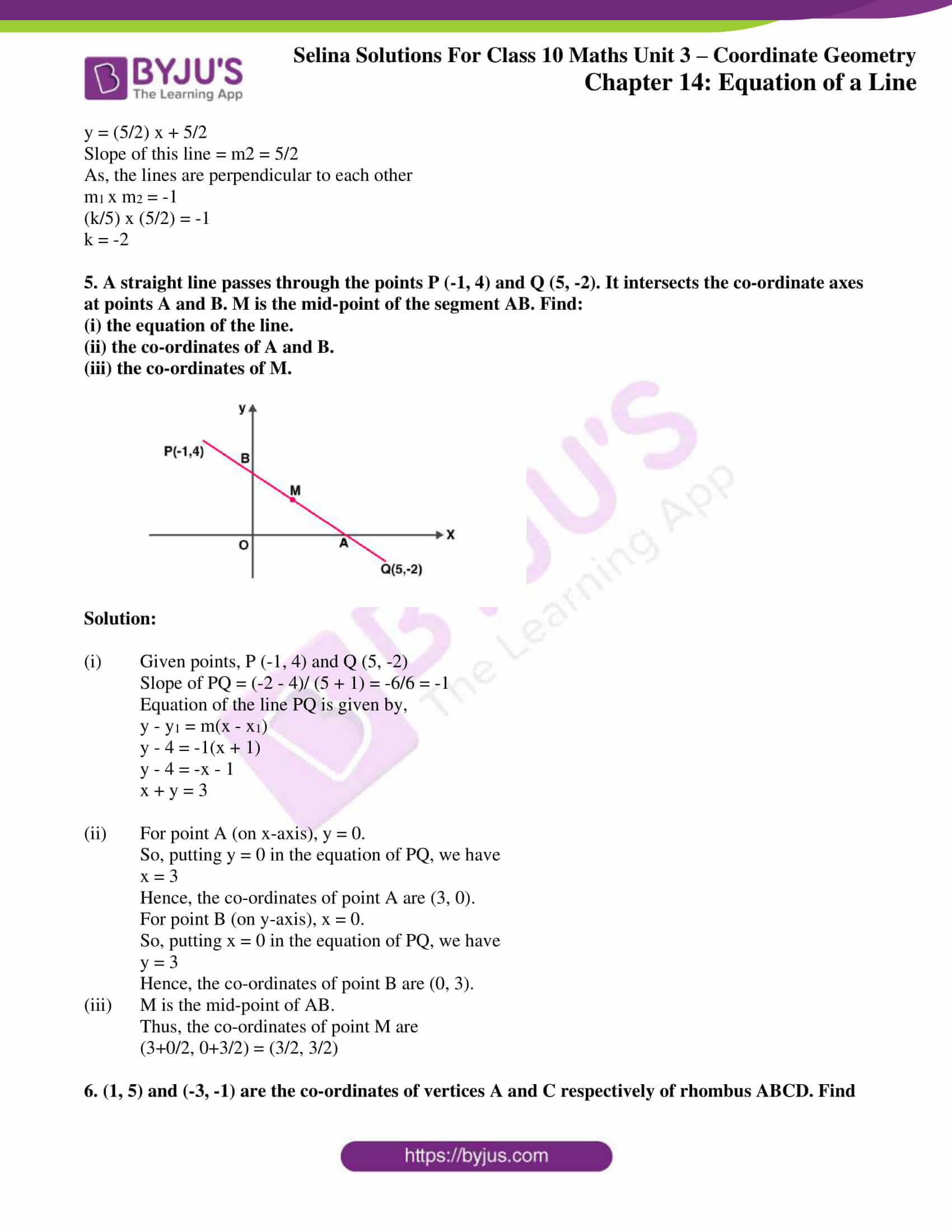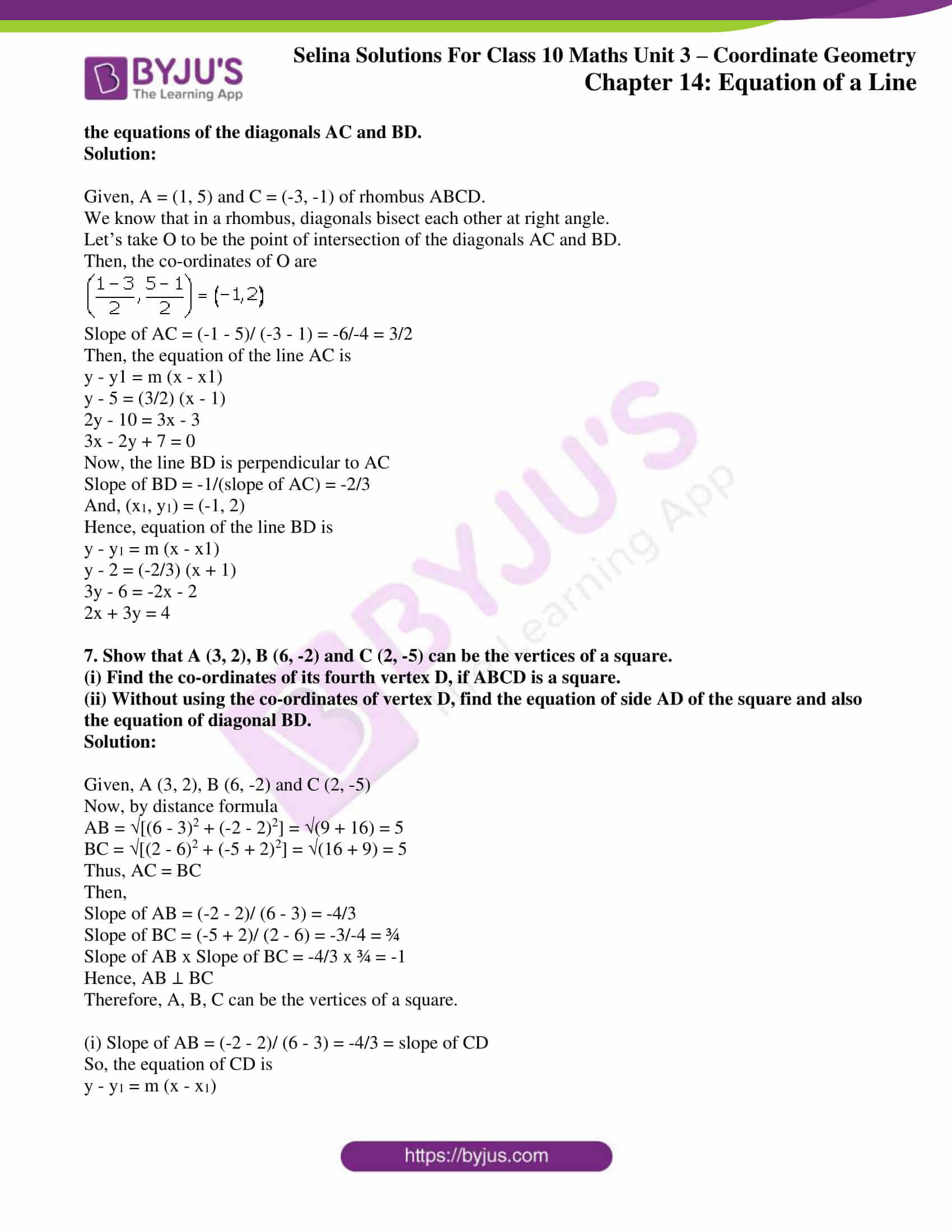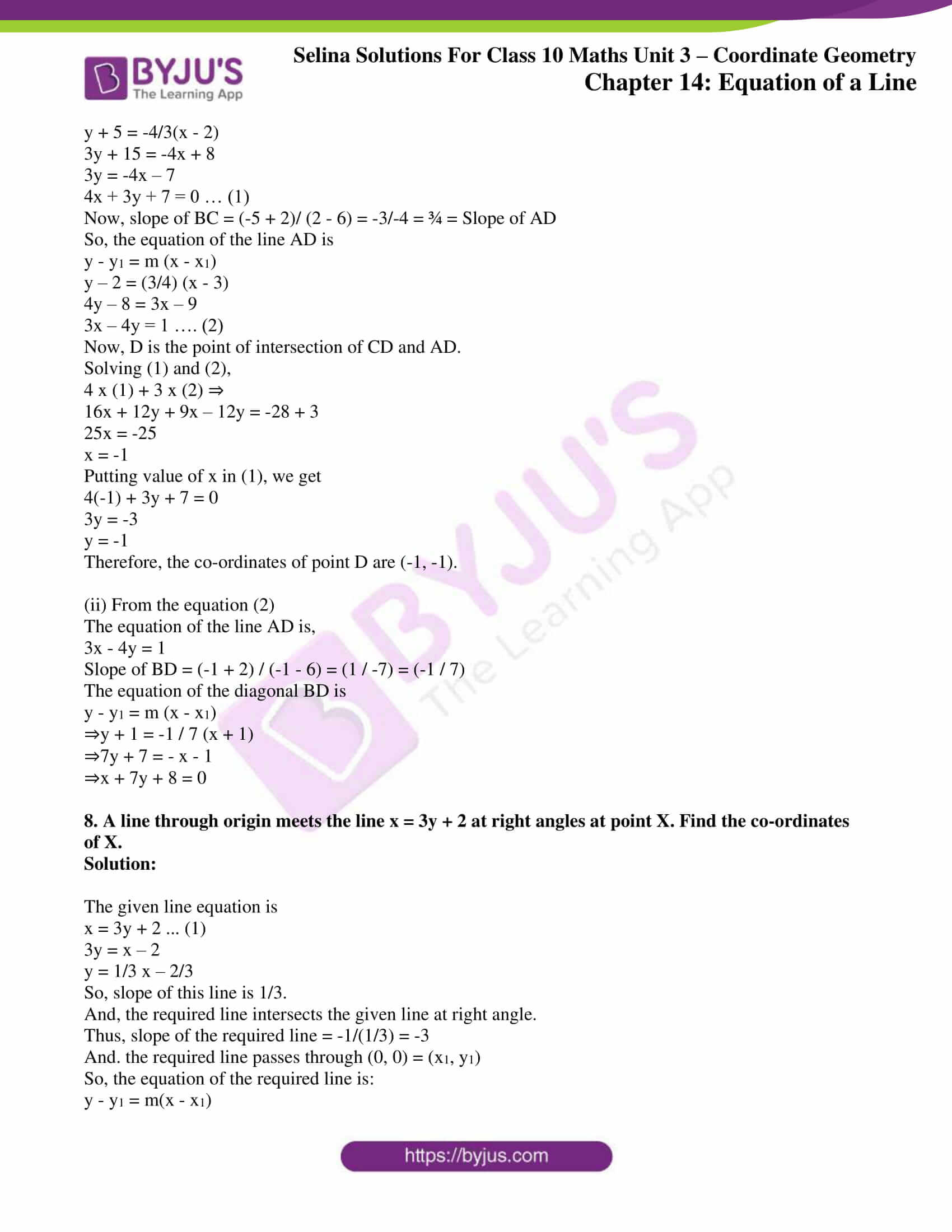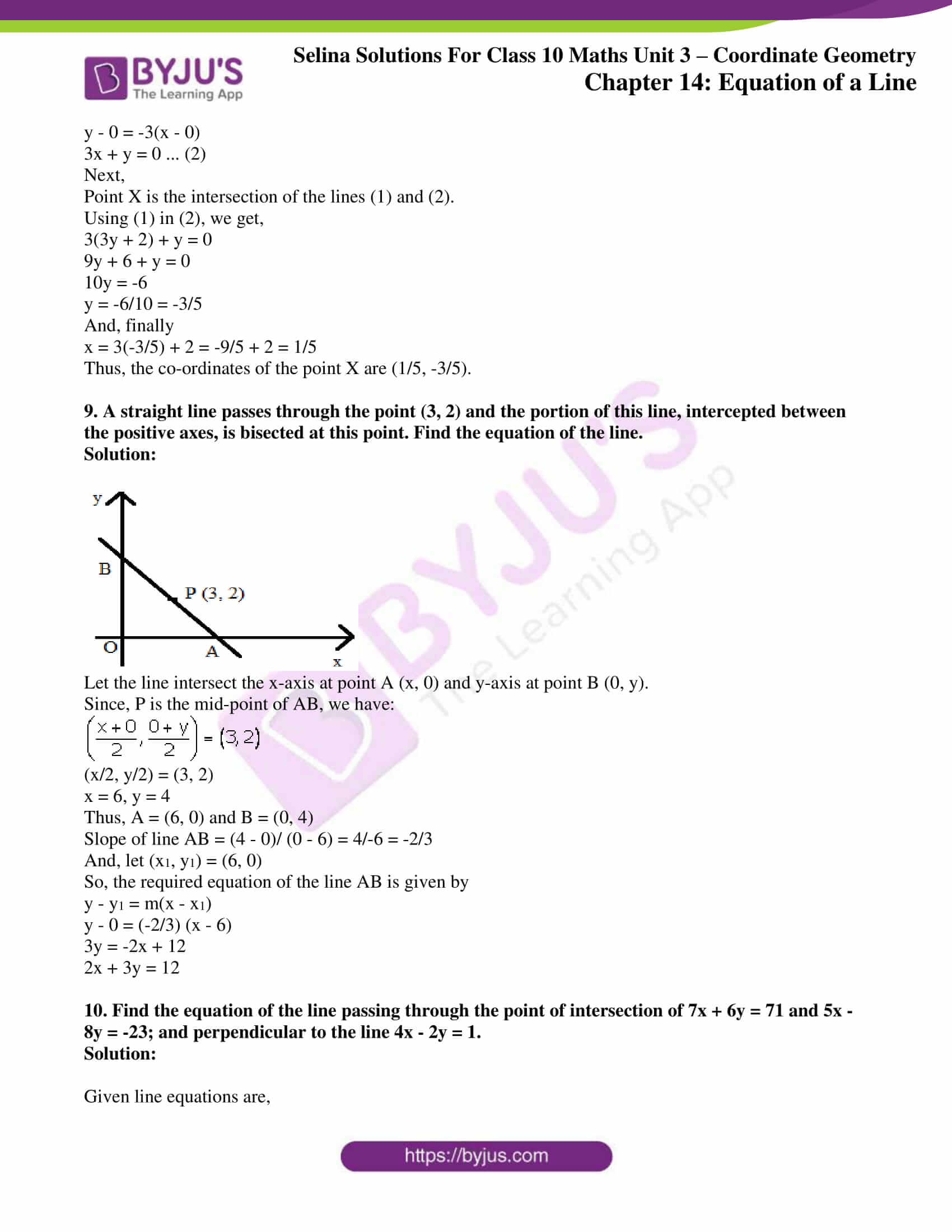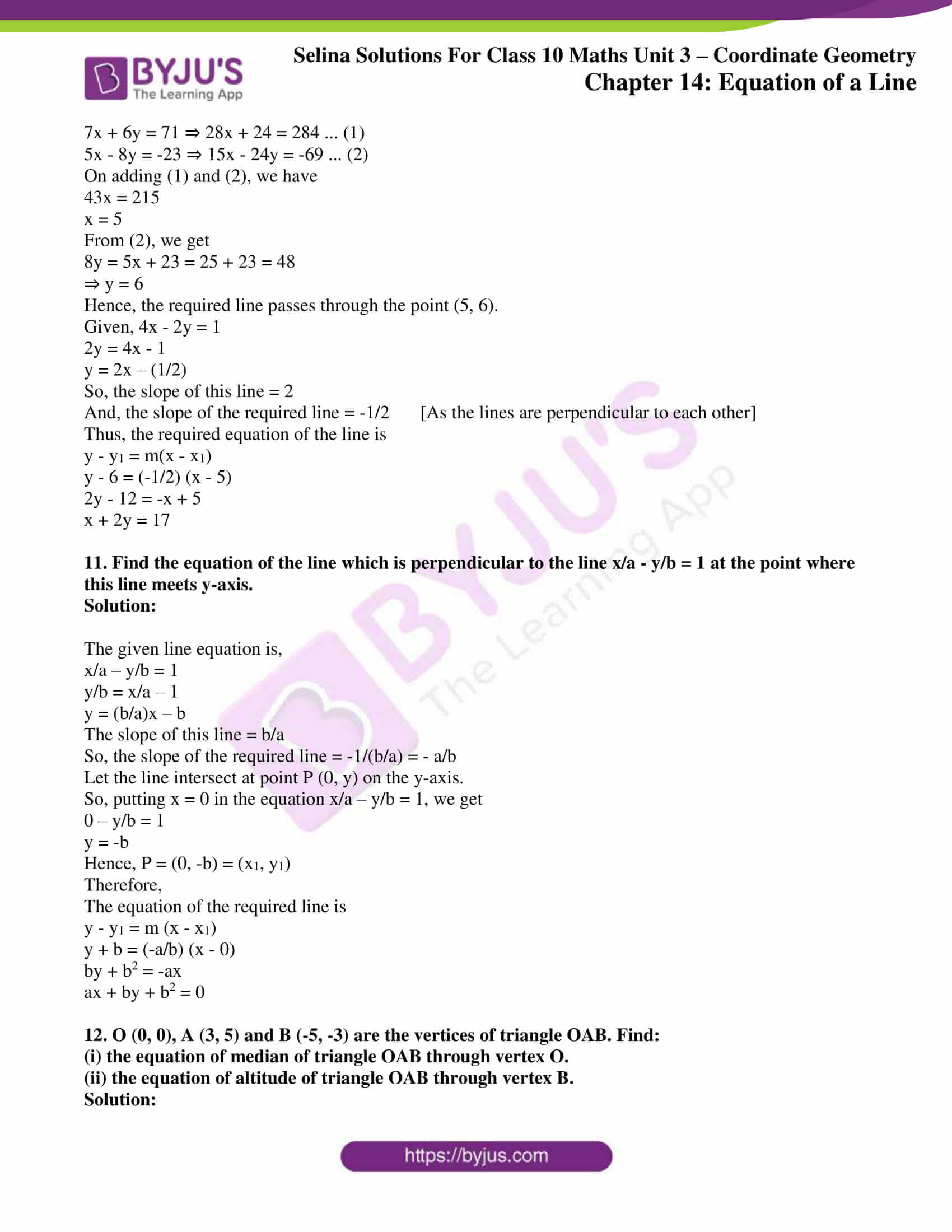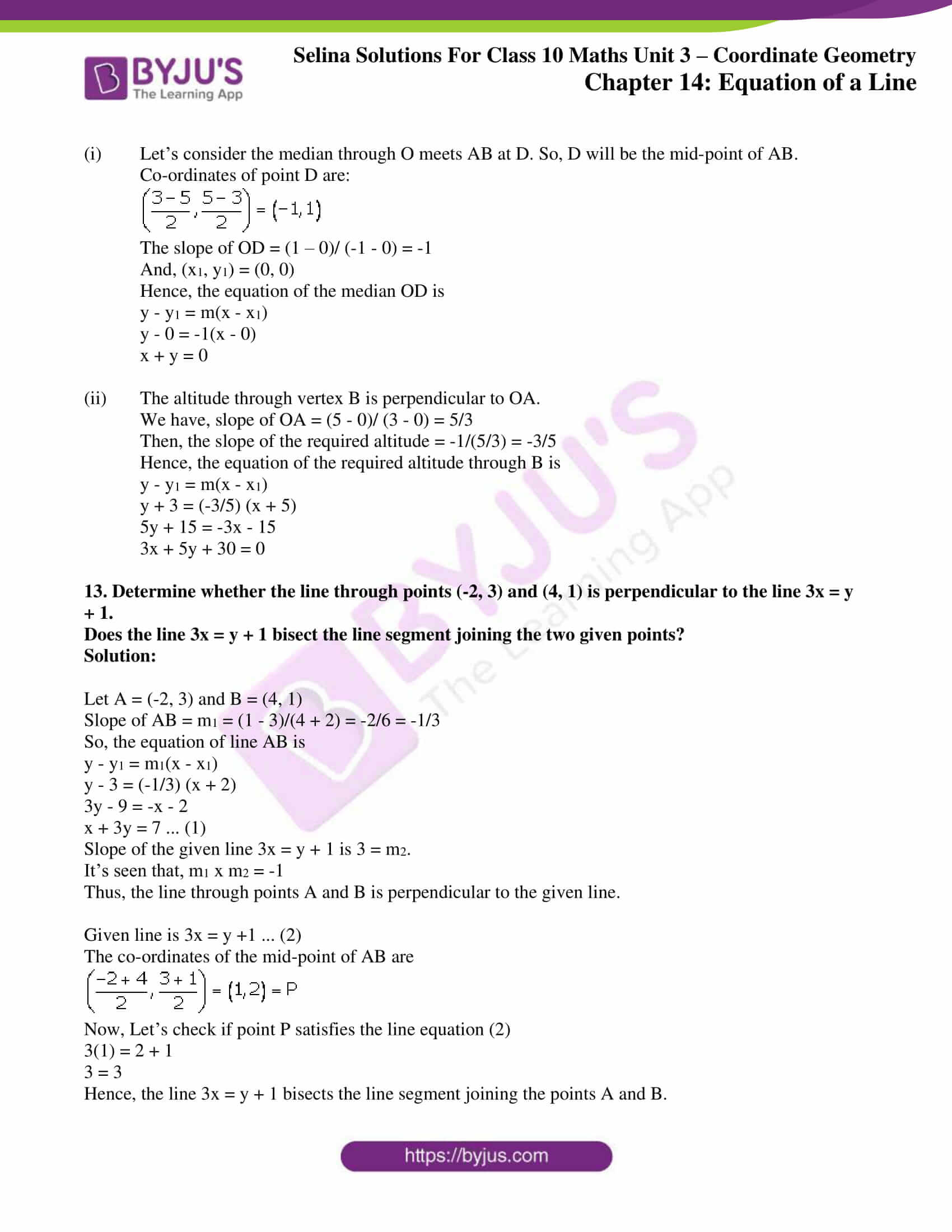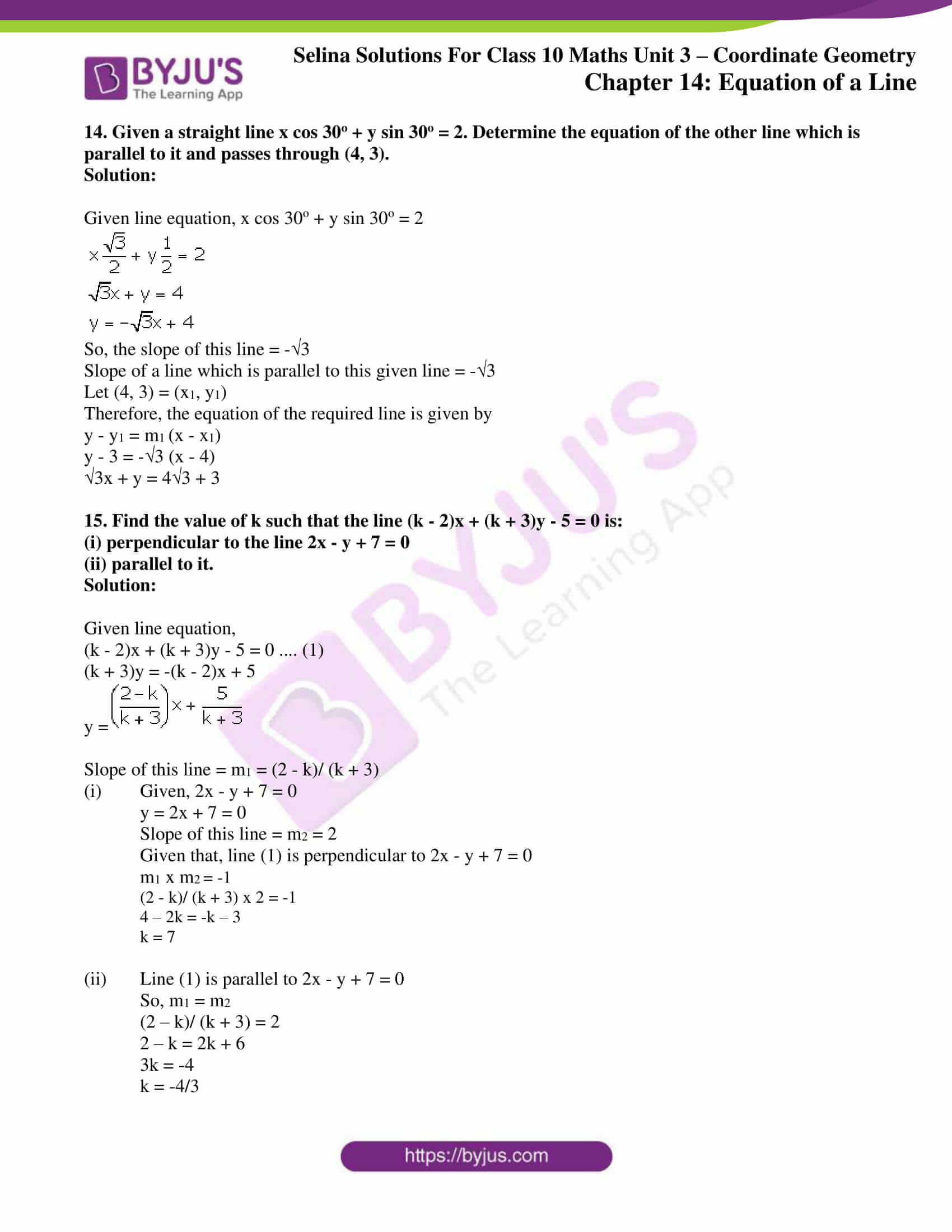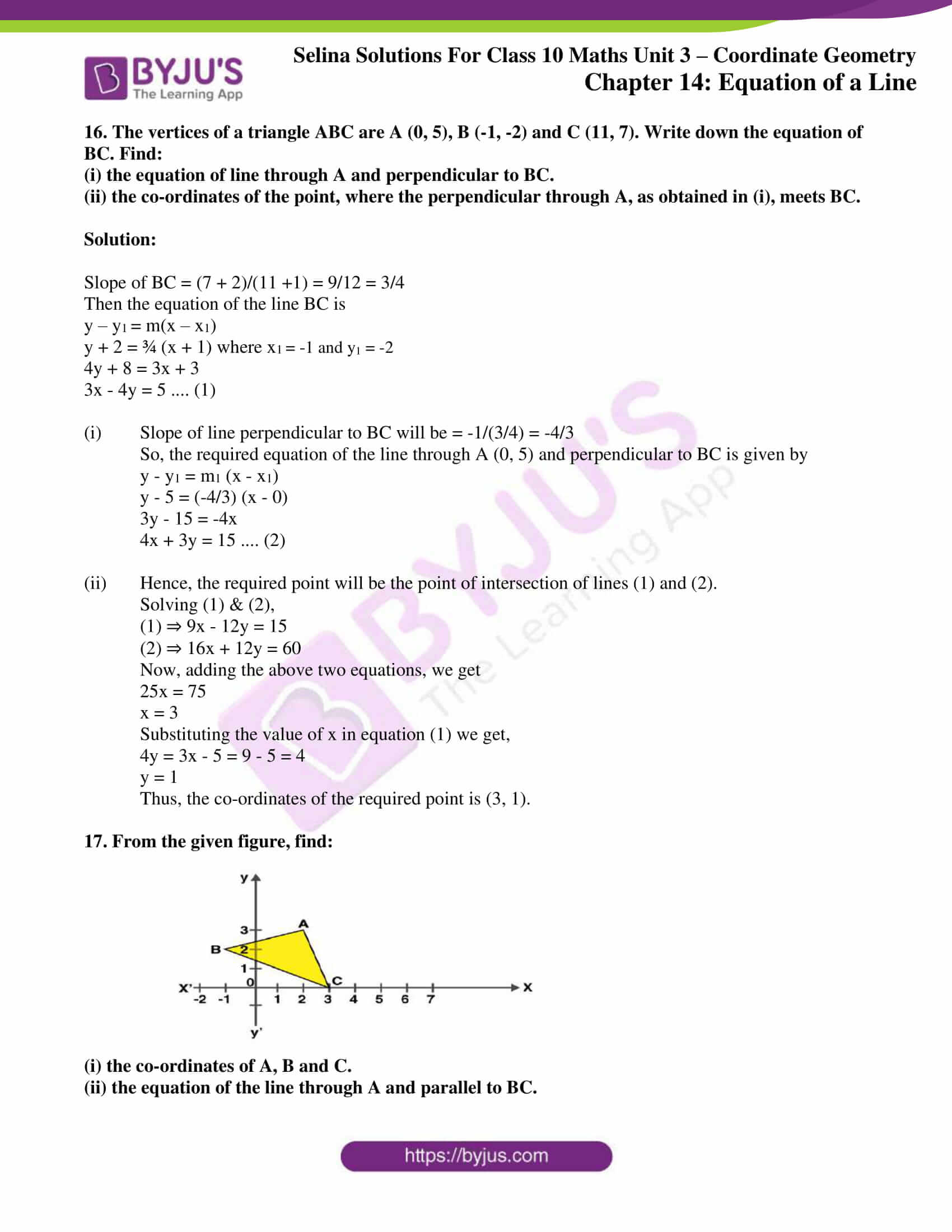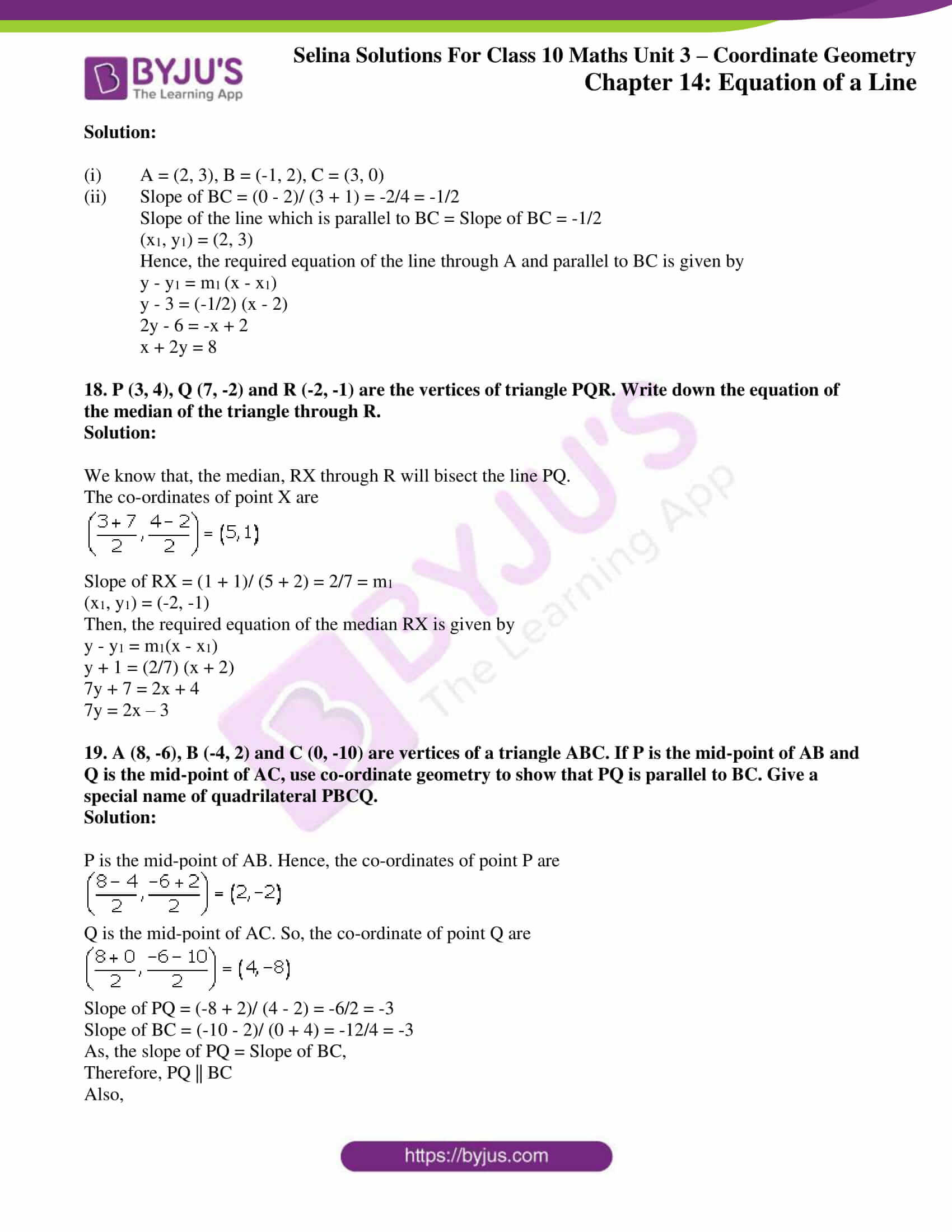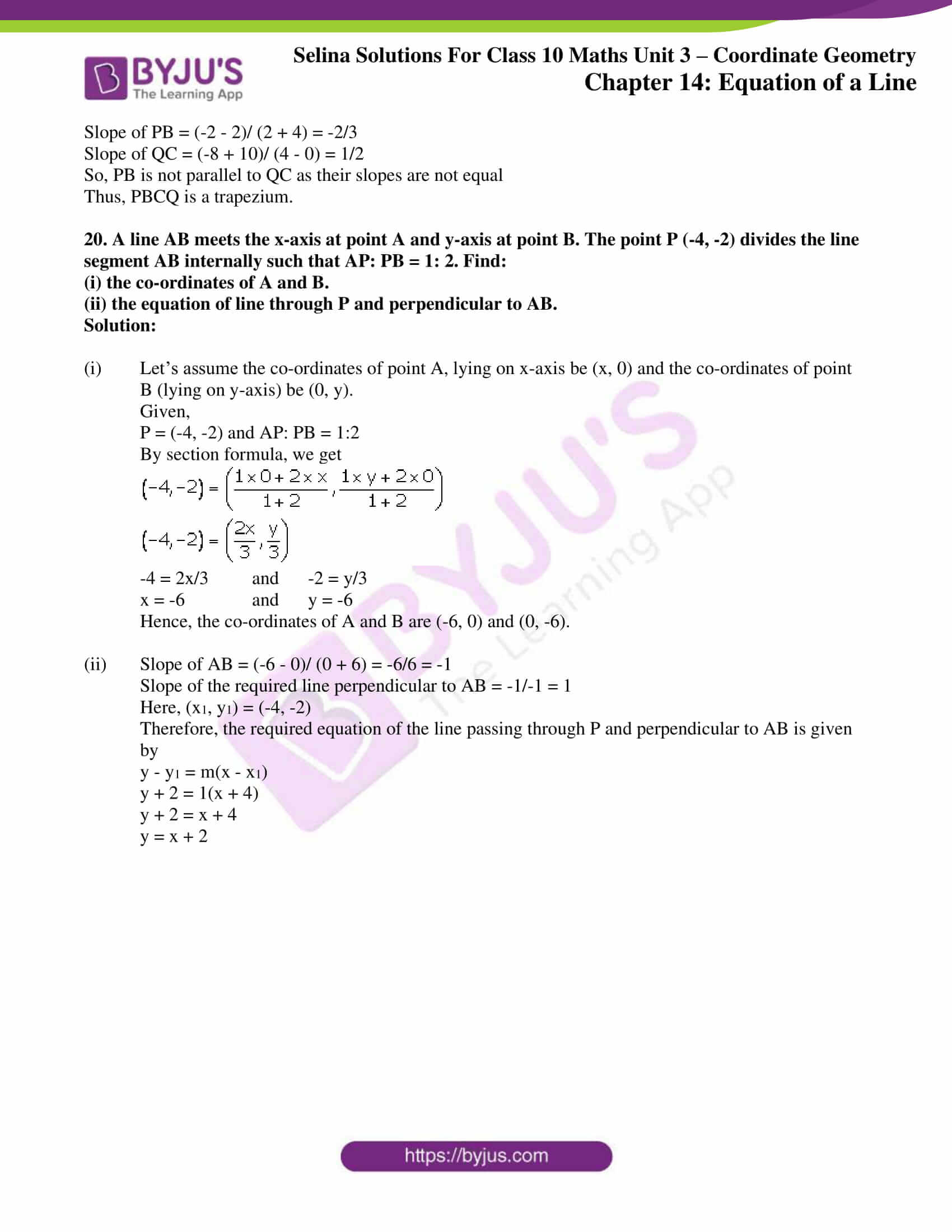### Access other exercises of Selina Solutions Concise Maths Class 10 Chapter 14 Equation of a Line

Exercise 14(A) Solutions

Exercise 14(B) Solutions

Exercise 14(C) Solutions

Exercise 14(D) Solutions

### Access Selina Solutions Concise Maths Class 10 Chapter 14 Equation of a Line Exercise 14(E)

#### Exercise 14(E) Page No: 202

1. Point P divides the line segment joining the points A (8, 0) and B (16, -8) in the ratio 3: 5. Find its co-ordinates of point P.

Also, find the equation of the line through P and parallel to 3x + 5y = 7.

Solution:

Given points, A (8, 0) and B (16, -8)

By section formula, the co-ordinates of the point P which divides AB in the ratio 3: 5 is given by= (11, -3) = (x1, y1)

Given line equation is,

3x + 5y = 7

5y = -3x + 7

y = (-3/5) x + 7/5

So, the slope of this line = -3/5

The line parallel to the line 3x + 5y = 7 will have the same slope,

Hence, the slope of the required line = Slope of the given line = -3/5

Thus,

The equation of the required line is

y – y1 = m(x – x1)

y + 3 = (-3/5)(x – 11)

5y + 15 = -3x + 33

3x + 5y = 18

2. The line segment joining the points A(3, -4) and B (-2, 1) is divided in the ratio 1: 3 at point P in it. Find the co-ordinates of P. Also, find the equation of the line through P and perpendicular to the line 5x – 3y + 4 = 0.

Solution:

Given points, A (3, -4) and B (-2, 1)

By section formula, the co-ordinates of the point P which divides AB in the ratio 1: 3 is given by= (7/4, -11/4) = (x1, y1)

Given line equation is,

5x – 3y + 4 = 0

3y = 5x + 4

y = (5/3) x + 4/3

So, the slope of this line = 5/3

The line perpendicular to the given line will have slope

Slope of the required line = -1/(5/3) = -3/5

Hence,

The equation of the required line is given by

y – y1 = m(x – x1)20y + 55 = -12x + 21

12x + 20y + 34 = 0

6x + 10y + 17 = 0

3. A line 5x + 3y + 15 = 0 meets y-axis at point P. Find the co-ordinates of point P. Find the equation of a line through P and perpendicular to x – 3y + 4 = 0.

Solution:

As the point P lies on y-axis,

Putting x = 0 in the equation 5x + 3y + 15 = 0, we get

5(0) + 3y + 15 = 0

y = -5

Hence, the co-ordinates of the point P are (0, -5).

Given line equation,

x – 3y + 4 = 0

3y = x + 4

y = (1/3)x + 4/3

Slope of this line = 1/3

From the question, the required line equation is perpendicular to the given equation: x – 3y + 4 = 0.

So, the product of their slopes is -1.

Slope of the required line = -1/(1/3) = -3

And,

(x1, y1) = (0, -5)

Therefore,

The required line equation is

y – y1 = m(x – x1)

y + 5 = -3(x – 0)

3x + y + 5 = 0

4. Find the value of k for which the lines kx – 5y + 4 = 0 and 5x – 2y + 5 = 0 are perpendicular to each other.

Solution:

Given,

kx – 5y + 4 = 0

5y = kx + 4

⇒ y = (k/5)x + 4/5

So, the slope of this line = m1 = k/5

And, for 5x – 2y + 5 = 0

⇒ 2y = 5x + 5

y = (5/2) x + 5/2

Slope of this line = m2 = 5/2

As, the lines are perpendicular to each other

m1 x m2 = -1

(k/5) x (5/2) = -1

k = -2

5. A straight line passes through the points P (-1, 4) and Q (5, -2). It intersects the co-ordinate axes at points A and B. M is the mid-point of the segment AB. Find:

(i) the equation of the line.

(ii) the co-ordinates of A and B.

(iii) the co-ordinates of M.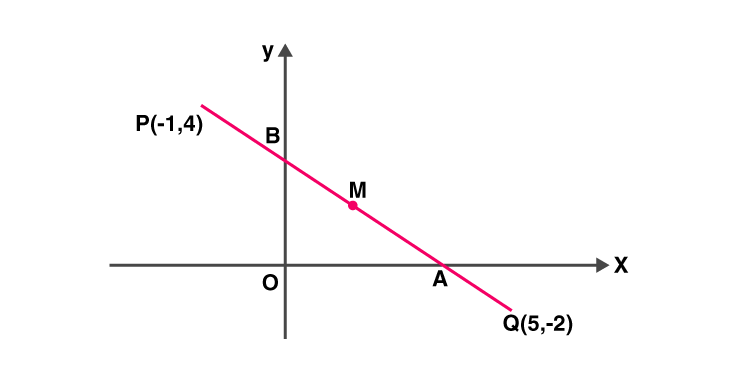Solution:

(i) Given points, P (-1, 4) and Q (5, -2)

Slope of PQ = (-2 – 4)/ (5 + 1) = -6/6 = -1

Equation of the line PQ is given by,

y – y1 = m(x – x1)

y – 4 = -1(x + 1)

y – 4 = -x – 1

x + y = 3

(ii) For point A (on x-axis), y = 0.

So, putting y = 0 in the equation of PQ, we have

x = 3

Hence, the co-ordinates of point A are (3, 0).

For point B (on y-axis), x = 0.

So, putting x = 0 in the equation of PQ, we have

y = 3

Hence, the co-ordinates of point B are (0, 3).

(iii) M is the mid-point of AB.

Thus, the co-ordinates of point M are

(3+0/2, 0+3/2) = (3/2, 3/2)

6. (1, 5) and (-3, -1) are the co-ordinates of vertices A and C respectively of rhombus ABCD. Find the equations of the diagonals AC and BD.

Solution:

Given, A = (1, 5) and C = (-3, -1) of rhombus ABCD.

We know that in a rhombus, diagonals bisect each other at right angle.

Let’s take O to be the point of intersection of the diagonals AC and BD.

Then, the co-ordinates of O are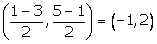Slope of AC = (-1 – 5)/ (-3 – 1) = -6/-4 = 3/2

Then, the equation of the line AC is

y – y1 = m (x – x1)

y – 5 = (3/2) (x – 1)

2y – 10 = 3x – 3

3x – 2y + 7 = 0

Now, the line BD is perpendicular to AC

Slope of BD = -1/(slope of AC) = -2/3

And, (x1, y1) = (-1, 2)

Hence, equation of the line BD is

y – y1 = m (x – x1)

y – 2 = (-2/3) (x + 1)

3y – 6 = -2x – 2

2x + 3y = 4

7. Show that A (3, 2), B (6, -2) and C (2, -5) can be the vertices of a square.

(i) Find the co-ordinates of its fourth vertex D, if ABCD is a square.

(ii) Without using the co-ordinates of vertex D, find the equation of side AD of the square and also the equation of diagonal BD.

Solution:

Given, A (3, 2), B (6, -2) and C (2, -5)

Now, by distance formula

AB = √[(6 – 3)2 + (-2 – 2)2] = √(9 + 16) = 5

BC = √[(2 – 6)2 + (-5 + 2)2] = √(16 + 9) = 5

Thus, AC = BC

Then,

Slope of AB = (-2 – 2)/ (6 – 3) = -4/3

Slope of BC = (-5 + 2)/ (2 – 6) = -3/-4 = ¾

Slope of AB x Slope of BC = -4/3 x ¾ = -1

Hence, AB ⊥ BC

Therefore, A, B, C can be the vertices of a square.

(i) Slope of AB = (-2 – 2)/ (6 – 3) = -4/3 = slope of CD

So, the equation of CD is

y – y1 = m (x – x1)

y + 5 = -4/3(x – 2)

3y + 15 = -4x + 8

3y = -4x – 7

4x + 3y + 7 = 0 … (1)

Now, slope of BC = (-5 + 2)/ (2 – 6) = -3/-4 = ¾ = Slope of AD

So, the equation of the line AD is

y – y1 = m (x – x1)

y – 2 = (3/4) (x – 3)

4y – 8 = 3x – 9

3x – 4y = 1 …. (2)

Now, D is the point of intersection of CD and AD.

Solving (1) and (2),

4 x (1) + 3 x (2) ⇒

16x + 12y + 9x – 12y = -28 + 3

25x = -25

x = -1

Putting value of x in (1), we get

4(-1) + 3y + 7 = 0

3y = -3

y = -1

Therefore, the co-ordinates of point D are (-1, -1).

(ii) From the equation (2)

The equation of the line AD is,

3x – 4y = 1

Slope of BD = (-1 + 2) / (-1 – 6) = (1 / -7) = (-1 / 7)

The equation of the diagonal BD is

y – y1 = m (x – x1)

⇒y + 1 = -1 / 7 (x + 1)

⇒7y + 7 = – x – 1

⇒x + 7y + 8 = 0

8. A line through origin meets the line x = 3y + 2 at right angles at point X. Find the co-ordinates of X.

Solution:

The given line equation is

x = 3y + 2 … (1)

3y = x – 2

y = 1/3 x – 2/3

So, slope of this line is 1/3.

And, the required line intersects the given line at right angle.

Thus, slope of the required line = -1/(1/3) = -3

And. the required line passes through (0, 0) = (x1, y1)

So, the equation of the required line is:

y – y1 = m(x – x1)

y – 0 = -3(x – 0)

3x + y = 0 … (2)

Next,

Point X is the intersection of the lines (1) and (2).

Using (1) in (2), we get,

3(3y + 2) + y = 0

9y + 6 + y = 0

10y = -6

y = -6/10 = -3/5

And, finally

x = 3(-3/5) + 2 = -9/5 + 2 = 1/5

Thus, the co-ordinates of the point X are (1/5, -3/5).

9. A straight line passes through the point (3, 2) and the portion of this line, intercepted between the positive axes, is bisected at this point. Find the equation of the line.

Solution: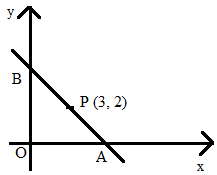Let the line intersect the x-axis at point A (x, 0) and y-axis at point B (0, y).

Since, P is the mid-point of AB, we have:(x/2, y/2) = (3, 2)

x = 6, y = 4

Thus, A = (6, 0) and B = (0, 4)

Slope of line AB = (4 – 0)/ (0 – 6) = 4/-6 = -2/3

And, let (x1, y1) = (6, 0)

So, the required equation of the line AB is given by

y – y1 = m(x – x1)

y – 0 = (-2/3) (x – 6)

3y = -2x + 12

2x + 3y = 12

10. Find the equation of the line passing through the point of intersection of 7x + 6y = 71 and 5x – 8y = -23; and perpendicular to the line 4x – 2y = 1.

Solution:

Given line equations are,

7x + 6y = 71 ⇒ 28x + 24 = 284 … (1)

5x – 8y = -23 ⇒ 15x – 24y = -69 … (2)

On adding (1) and (2), we have

43x = 215

x = 5

From (2), we get

8y = 5x + 23 = 25 + 23 = 48

⇒ y = 6

Hence, the required line passes through the point (5, 6).

Given, 4x – 2y = 1

2y = 4x – 1

y = 2x – (1/2)

So, the slope of this line = 2

And, the slope of the required line = -1/2 [As the lines are perpendicular to each other]

Thus, the required equation of the line is

y – y1 = m(x – x1)

y – 6 = (-1/2) (x – 5)

2y – 12 = -x + 5

x + 2y = 17

11. Find the equation of the line which is perpendicular to the line x/a – y/b = 1 at the point where this line meets y-axis.

Solution:

The given line equation is,

x/a – y/b = 1

y/b = x/a – 1

y = (b/a)x – b

The slope of this line = b/a

So, the slope of the required line = -1/(b/a) = – a/b

Let the line intersect at point P (0, y) on the y-axis.

So, putting x = 0 in the equation x/a – y/b = 1, we get

0 – y/b = 1

y = -b

Hence, P = (0, -b) = (x1, y1)

Therefore,

The equation of the required line is

y – y1 = m (x – x1)

y + b = (-a/b) (x – 0)

by + b2 = -ax

ax + by + b2 = 0

12. O (0, 0), A (3, 5) and B (-5, -3) are the vertices of triangle OAB. Find:

(i) the equation of median of triangle OAB through vertex O.

(ii) the equation of altitude of triangle OAB through vertex B.

Solution:

(i) Let’s consider the median through O meets AB at D. So, D will be the mid-point of AB.

Co-ordinates of point D are: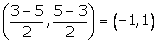The slope of OD = (1 – 0)/ (-1 – 0) = -1

And, (x1, y1) = (0, 0)

Hence, the equation of the median OD is

y – y1 = m(x – x1)

y – 0 = -1(x – 0)

x + y = 0

(ii) The altitude through vertex B is perpendicular to OA.

We have, slope of OA = (5 – 0)/ (3 – 0) = 5/3

Then, the slope of the required altitude = -1/(5/3) = -3/5

Hence, the equation of the required altitude through B is

y – y1 = m(x – x1)

y + 3 = (-3/5) (x + 5)

5y + 15 = -3x – 15

3x + 5y + 30 = 0

13. Determine whether the line through points (-2, 3) and (4, 1) is perpendicular to the line 3x = y + 1.

Does the line 3x = y + 1 bisect the line segment joining the two given points?

Solution:

Let A = (-2, 3) and B = (4, 1)

Slope of AB = m1 = (1 – 3)/(4 + 2) = -2/6 = -1/3

So, the equation of line AB is

y – y1 = m1(x – x1)

y – 3 = (-1/3) (x + 2)

3y – 9 = -x – 2

x + 3y = 7 … (1)

Slope of the given line 3x = y + 1 is 3 = m2.

It’s seen that, m1 x m2 = -1

Thus, the line through points A and B is perpendicular to the given line.

Given line is 3x = y +1 … (2)

The co-ordinates of the mid-point of AB are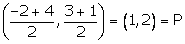Now, Let’s check if point P satisfies the line equation (2)

3(1) = 2 + 1

3 = 3

Hence, the line 3x = y + 1 bisects the line segment joining the points A and B.

14. Given a straight line x cos 30o + y sin 30o = 2. Determine the equation of the other line which is parallel to it and passes through (4, 3).

Solution:

Given line equation, x cos 30o + y sin 30o = 2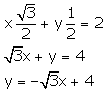So, the slope of this line = -√3

Slope of a line which is parallel to this given line = -√3

Let (4, 3) = (x1, y1)

Therefore, the equation of the required line is given by

y – y1 = m1 (x – x1)

y – 3 = -√3 (x – 4)

√3x + y = 4√3 + 3

15. Find the value of k such that the line (k – 2)x + (k + 3)y – 5 = 0 is:

(i) perpendicular to the line 2x – y + 7 = 0

(ii) parallel to it.

Solution:

Given line equation,

(k – 2)x + (k + 3)y – 5 = 0 …. (1)

(k + 3)y = -(k – 2)x + 5

y =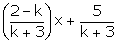Slope of this line = m1 = (2 – k)/ (k + 3)

(i) Given, 2x – y + 7 = 0

y = 2x + 7 = 0

Slope of this line = m2 = 2

Given that, line (1) is perpendicular to 2x – y + 7 = 0

m1 x m2 = -1

(2 – k)/ (k + 3) x 2 = -1

4 – 2k = -k – 3

k = 7

(ii) Line (1) is parallel to 2x – y + 7 = 0

So, m1 = m2

(2 – k)/ (k + 3) = 2

2 – k = 2k + 6

3k = -4

k = -4/3

16. The vertices of a triangle ABC are A (0, 5), B (-1, -2) and C (11, 7). Write down the equation of BC. Find:

(i) the equation of line through A and perpendicular to BC.

(ii) the co-ordinates of the point, where the perpendicular through A, as obtained in (i), meets BC.

Solution:

Slope of BC = (7 + 2)/(11 +1) = 9/12 = 3/4

Then the equation of the line BC is

y – y1 = m(x – x1)

y + 2 = ¾ (x + 1) where x1 = -1 and y1 = -2

4y + 8 = 3x + 3

3x – 4y = 5 …. (1)

(i) Slope of line perpendicular to BC will be = -1/(3/4) = -4/3

So, the required equation of the line through A (0, 5) and perpendicular to BC is given by

y – y1 = m1 (x – x1)

y – 5 = (-4/3) (x – 0)

3y – 15 = -4x

4x + 3y = 15 …. (2)

(ii) Hence, the required point will be the point of intersection of lines (1) and (2).

Solving (1) & (2),

(1) ⇒ 9x – 12y = 15

(2) ⇒ 16x + 12y = 60

Now, adding the above two equations, we get

25x = 75

x = 3

Substituting the value of x in equation (1) we get,

4y = 3x – 5 = 9 – 5 = 4

y = 1

Thus, the co-ordinates of the required point is (3, 1).

17. From the given figure, find: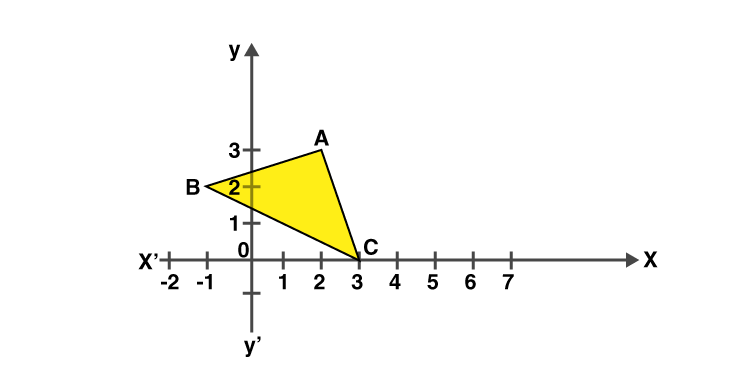(i) the co-ordinates of A, B and C.

(ii) the equation of the line through A and parallel to BC.

Solution:

(i) A = (2, 3), B = (-1, 2), C = (3, 0)

(ii) Slope of BC = (0 – 2)/ (3 + 1) = -2/4 = -1/2

Slope of the line which is parallel to BC = Slope of BC = -1/2

(x1, y1) = (2, 3)

Hence, the required equation of the line through A and parallel to BC is given by

y – y1 = m1 (x – x1)

y – 3 = (-1/2) (x – 2)

2y – 6 = -x + 2

x + 2y = 8

18. P (3, 4), Q (7, -2) and R (-2, -1) are the vertices of triangle PQR. Write down the equation of the median of the triangle through R.

Solution:

We know that, the median, RX through R will bisect the line PQ.

The co-ordinates of point X are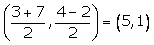Slope of RX = (1 + 1)/ (5 + 2) = 2/7 = m1

(x1, y1) = (-2, -1)

Then, the required equation of the median RX is given by

y – y1 = m1(x – x1)

y + 1 = (2/7) (x + 2)

7y + 7 = 2x + 4

7y = 2x – 3

19. A (8, -6), B (-4, 2) and C (0, -10) are vertices of a triangle ABC. If P is the mid-point of AB and Q is the mid-point of AC, use co-ordinate geometry to show that PQ is parallel to BC. Give a special name of quadrilateral PBCQ.

Solution:

P is the mid-point of AB. Hence, the co-ordinates of point P are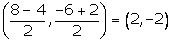Q is the mid-point of AC. So, the co-ordinate of point Q are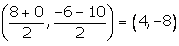Slope of PQ = (-8 + 2)/ (4 – 2) = -6/2 = -3

Slope of BC = (-10 – 2)/ (0 + 4) = -12/4 = -3

As, the slope of PQ = Slope of BC,

Therefore, PQ || BC

Also,

Slope of PB = (-2 – 2)/ (2 + 4) = -2/3

Slope of QC = (-8 + 10)/ (4 – 0) = 1/2

So, PB is not parallel to QC as their slopes are not equal

Thus, PBCQ is a trapezium.

20. A line AB meets the x-axis at point A and y-axis at point B. The point P (-4, -2) divides the line segment AB internally such that AP: PB = 1: 2. Find:

(i) the co-ordinates of A and B.

(ii) the equation of line through P and perpendicular to AB.

Solution:

(i) Let’s assume the co-ordinates of point A, lying on x-axis be (x, 0) and the co-ordinates of point B (lying on y-axis) be (0, y).

Given,

P = (-4, -2) and AP: PB = 1:2

By section formula, we get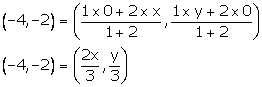-4 = 2x/3 and -2 = y/3

x = -6 and y = -6

Hence, the co-ordinates of A and B are (-6, 0) and (0, -6).

(ii) Slope of AB = (-6 – 0)/ (0 + 6) = -6/6 = -1

Slope of the required line perpendicular to AB = -1/-1 = 1

Here, (x1, y1) = (-4, -2)

Therefore, the required equation of the line passing through P and perpendicular to AB is given by

y – y1 = m(x – x1)

y + 2 = 1(x + 4)

y + 2 = x + 4

y = x + 2